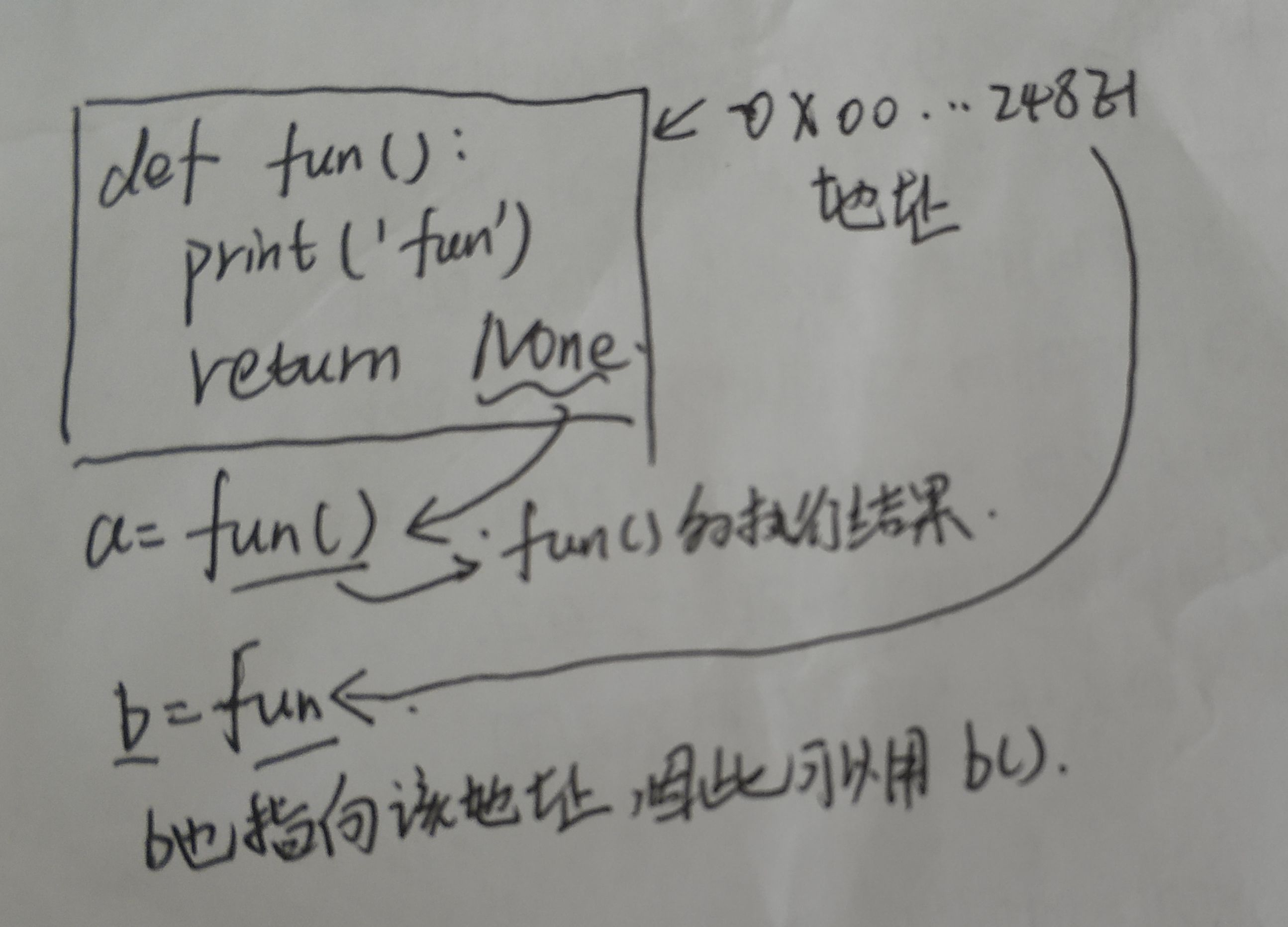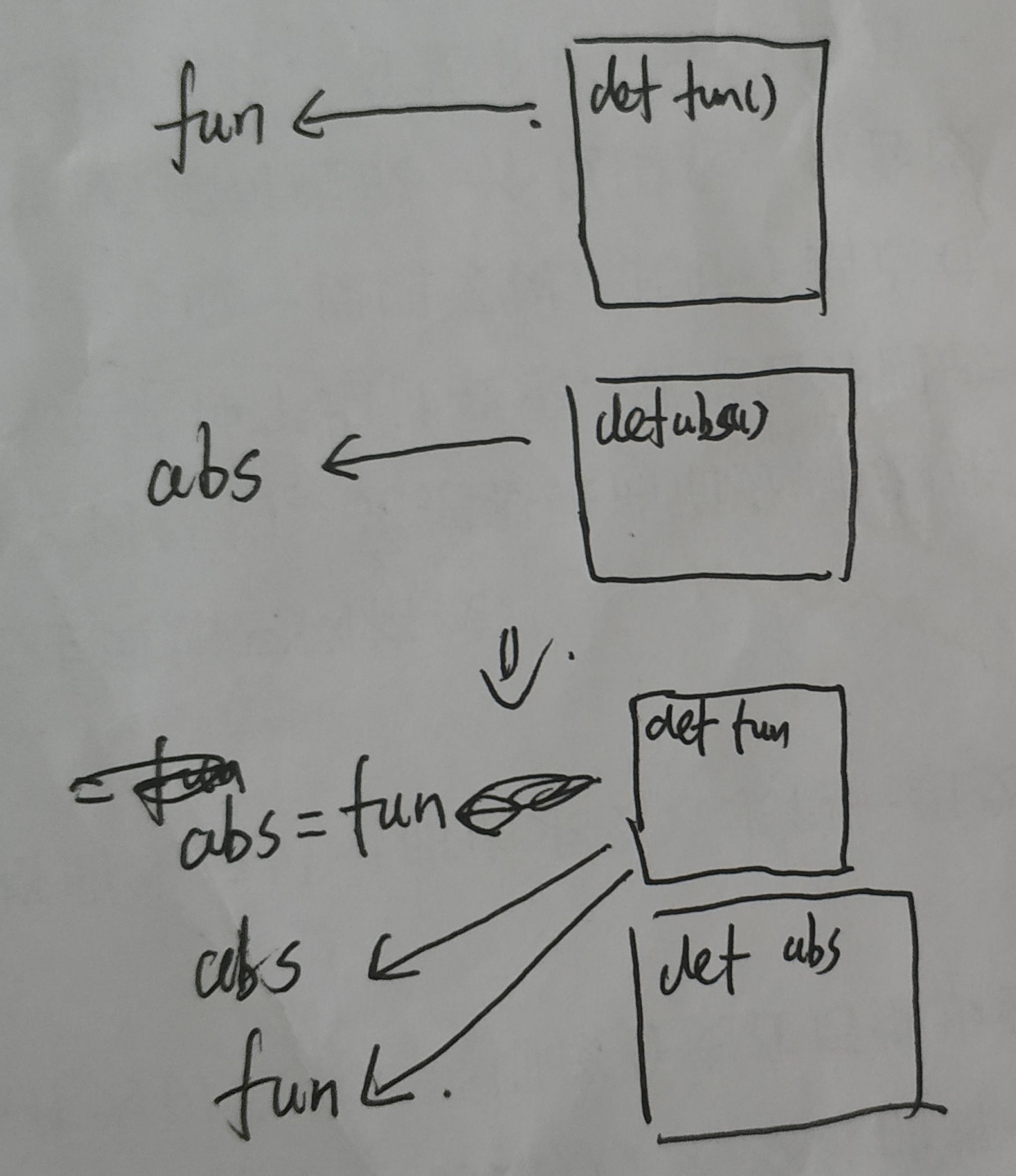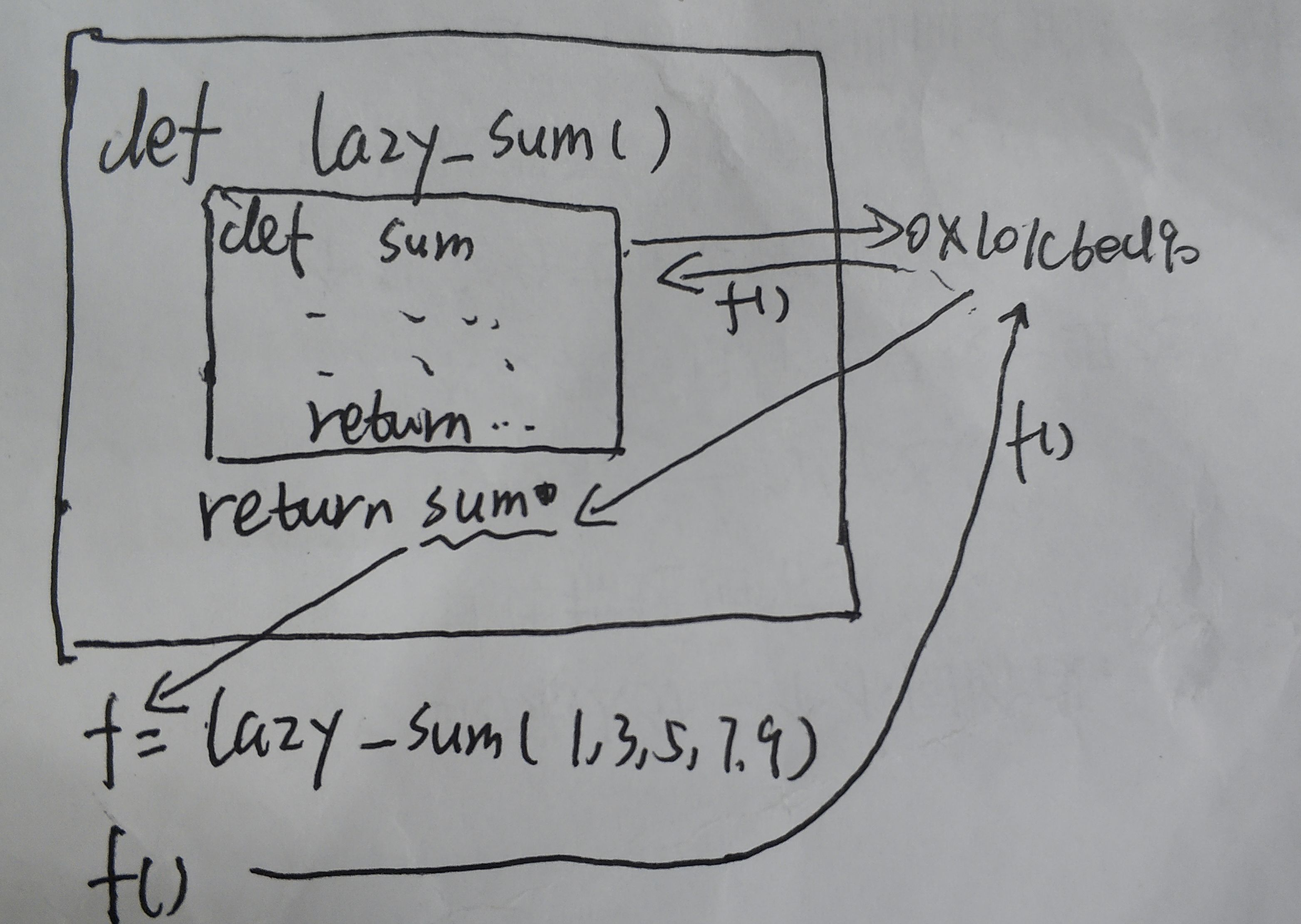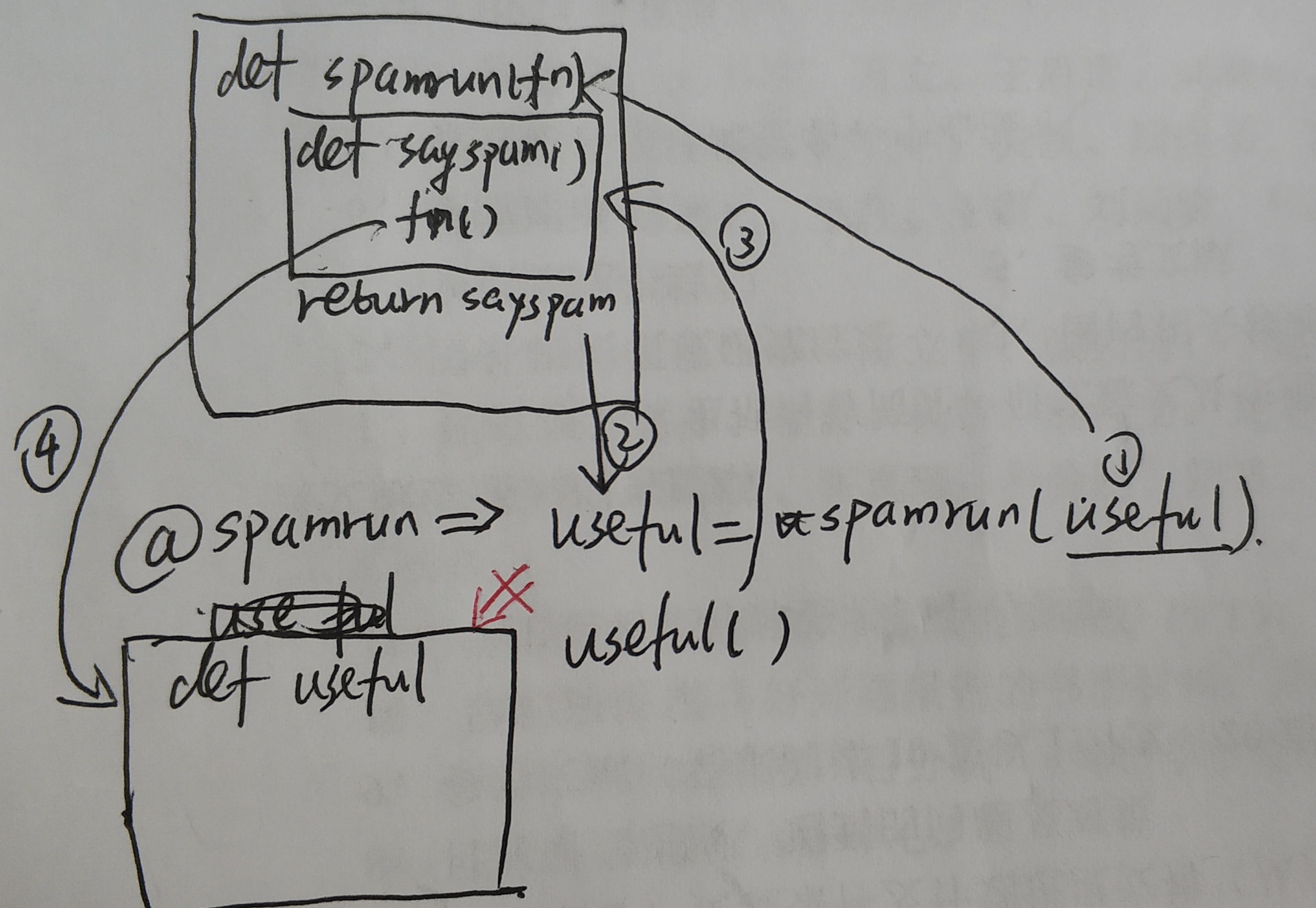TOP
Python中使用@的理解(一)
2019-07-11 16:09:45 】 浏览:845
 Tags：Python 使用 理解

# Python函数中使用@

## 稍提一下的基础

### `fun` 和`fun()`的区别

``````def fun():
print('fun')
return None

a = fun() #fun函数并将返回值给a
print('a的值为',a)
b = fun #将fun函数地址赋给b
b() #调用b，b和fun指向的地址相同
print('b的值为',b)
'''输出
fun
a的值为 None
fun
b的值为 <function fun at 0x00000248E1EBE0D0>
'''````````````>>>a = abs
>>>a(-1)
1``````

``````def fun():
print('fun')
abs = fun
abs() #输出fun
``````### 返回函数

``````def lazy_sum(*args):
def sum():
ax = 0
for n in args:
ax = ax + n
return ax
return sum
>>> f = lazy_sum(1, 3, 5, 7, 9)
>>> f
<function lazy_sum.<locals>.sum at 0x101c6ed90>
>>>f()
25````````````def lazy_sum(*args):
def sum():
ax = 0
for n in args:
ax = ax + n
return ax
return 1

f = lazy_sum(1,3,5,7,9)
print(f)#Q输出1

print(f())#报错'int' object is not callable
``````

?返回函数中的闭包问题也要了解一下，内嵌函数可以访问外层函数的变量

### 参数的嵌套调用

``````def lazy_sum():
def sum(*args):
ax = 0
for n in args:
ax = ax + n
return ax
return sum

f = lazy_sum()(1,3,5,7,9)
print(f)#输出25``````

1. 执行 `lazy_sum()`，返回`sum`;
2. 执行`sum(1,3,5,7,9)`，返回25;
3. `25`赋给`f`

## @的使用

### 不带参数的单一使用（一个@修饰）

``````def spamrun(fn):
def sayspam():
print("spam,spam,spam")
fn()
return sayspam
@spamrun
def useful():
print('useful')

useful()
'''

spam,spam,spam
useful
'''``````

1. 在初始化时，解释器读到`@spamrun`,此时将下方的`useful`作为参数传入到`spamrun`
2. `spamrun(useful)`中，由于是返回函数，直接将`sayspam()`的内存地址赋给`useful`
3. 执行`useful()`,此时`useful`指向了`sayspam`，因此打印`spam,spam,spam`。然后执行`fn()`，此时的`fn`才指向原来`useful()`的地址，开始执行`print('useful')```````def log(func):
def wrapper(*args, **kw):
print('call %s():' % func.__name__)
return func(*args, **kw)
return wrapper
@log
def now():
print('2015-3-25')

now()
'''
call now():
2015-3-25
'''``````

### 不带参数的多次使用（两个@）

``````def spamrun(fn):
def sayspam():
print("spam,spam,spam")
fn()
return sayspam

def spamrun1(fn):
def sayspam1():
print("spam1,spam1,spam1")
fn()
return sayspam1

@spamrun
@spamrun1
def useful():
print('useful')

useful()
'''
spam,spam,spam
spam1,spam1,spam1
useful
'''``````

? 1. 解释器从上到下读取，先读到`@spamrun`,于是向下查找第一个函数定义`useful()`，于是有`useful=spamrun(useful)`

? 2. 再读到`@spamrun1`，重复上次操作，但此时的变量`useful`已经被装饰过一次，再被装饰第二次，因此有`useful=spamrun1(useful)`

### 带参数的单次使用

``````def log(text):
def decorator(func):
def wrapper():
print('%s %s():' % (text, func.__name__))
return func()
return wrapper
return decorator

@log('execute')
def now():
Python中使用@的理解(一) https://www.cppentry.com/bencandy.php?fid=77&id=227528``````

】【打印繁体】【投稿】【收藏】 【推荐】【举报】【评论】 【关闭】 【返回顶部